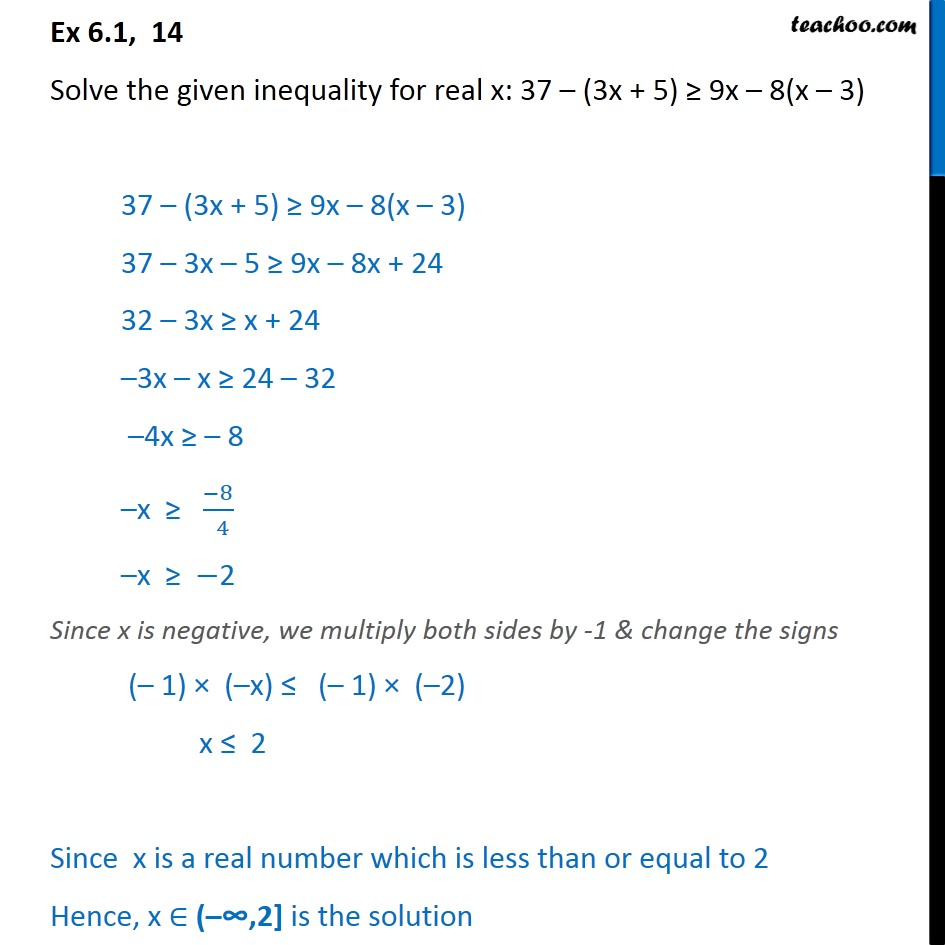Ex 6.1

Chapter 6 Class 11 Linear Inequalities (Term 2)
Serial order wise### Transcript

Ex 6.1, 14 Solve the given inequality for real x: 37 ­– (3x + 5) ≥ 9x – 8(x – 3) 37 ­– (3x + 5) ≥ 9x – 8(x – 3) 37 – 3x – 5 ≥ 9x – 8x + 24 32 – 3x ≥ x + 24 –3x – x ≥ 24 – 32 –4x ≥ – 8 –x ≥ ﷐−8﷮ 4﷯ –x ≥ −2 Since x is negative, we multiply both sides by -1 & change the signs (– 1) × (–x) ≤ (– 1) × (–2) x ≤ 2 Since x is a real number which is less than or equal to 2 Hence, x ∈ (–𕔴2] is the solution